Learning Center > Teaching with Sketchpad > Sample Activities > Trigonometry, Conics, and Precalculus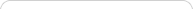##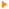Teaching with Sketchpad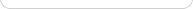•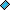- plays a video
•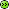- opens a web page# Trigonometry, Conics, and Precalculus

NOTE: If you are using Internet Explorer and are unable to download the .zip activity files, right-click the download link and choose Save Target As to save to your computer.

##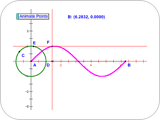A Sine Wave Tracer

Students use points on perpendiculars, parallels, and a circle to construct an animation that traces out a sine wave. They explore the sine wave by dragging various points in their construction. Finally, students explore the relationship between a unit circle, its circumference, and the trace of a sine wave.

##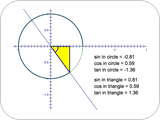Unit Circle and Right Triangle Functions

Students explore the relationships between the unit circle definitions of trigonometric functions and the right triangle definitions. They then combine the two models and examine the similarities and differences that emerge.

##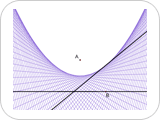Patty Paper Parabolas

Students fold a piece of paper so that the creases outline the shape of a parabola. They compare their parabolas with those of other students to see how the shape depends on the positions of the focus and directrix. Students then do the same construction with Sketchpad and explore how moving the focus or directrix changes the shape of the folds. Finally students are challenged to construct the parabola itself (not just the folds) and to prove that their construction matches the geometric locus definition of a parabola.

##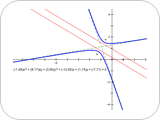Analytic Conics

Students explore conic sections analytically. They change parameters in the equation of a conic in standard position and observe changes in the graph, and then explore the general equation of a conic section.

##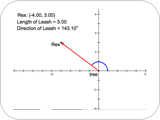Introduction to Vectors: Walking Rex

Students learn two ways to describe vectors, convert between the two descriptions, and move vectors around the plane to explore how vectors are independent of specific positions.

##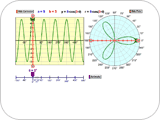Cartesian Graphs and Polar Graphs

Students compare rectangular graphs and polar graphs for functions in the form y = a sin(bx) and r = a sin(). They find connections between the two types of graphing when they analyze how the period and amplitude of a Cartesian graph correlate with features of the corresponding polar graph. They make predictions as to how changing a and b will affect the polar graph and then check their predictions.